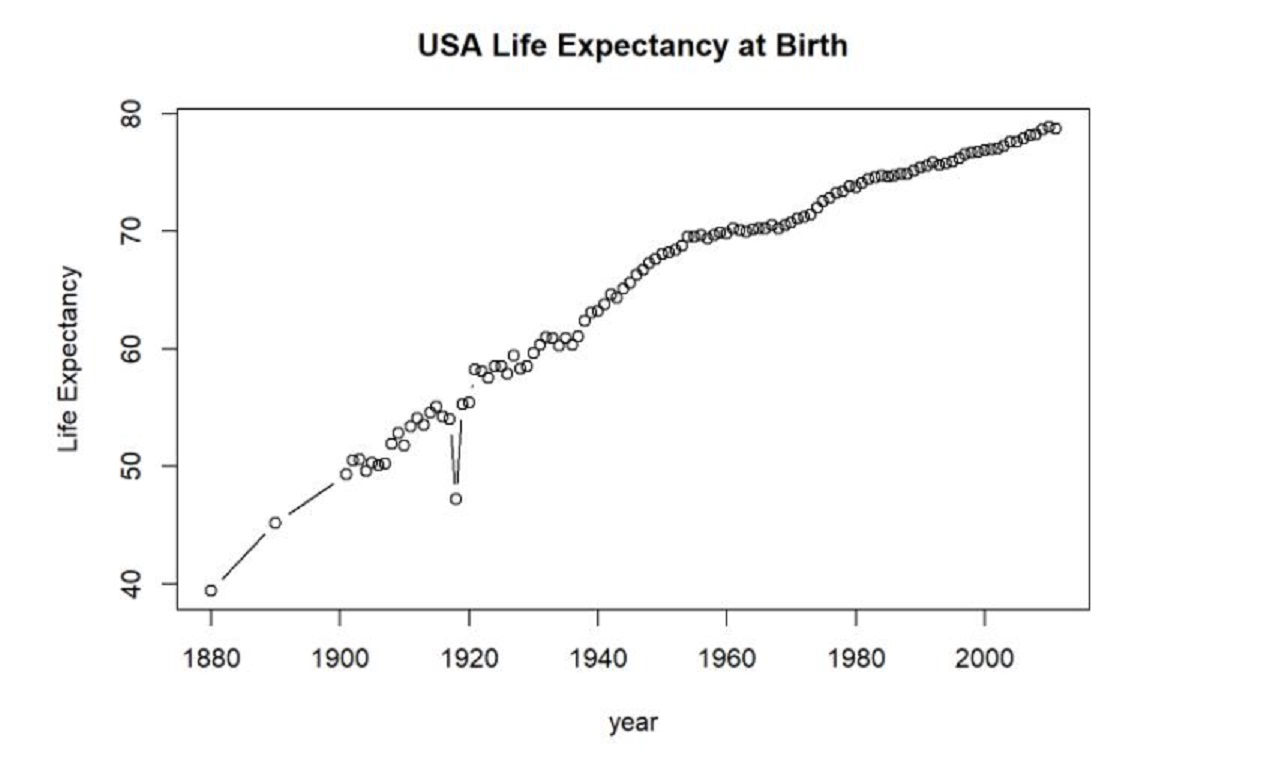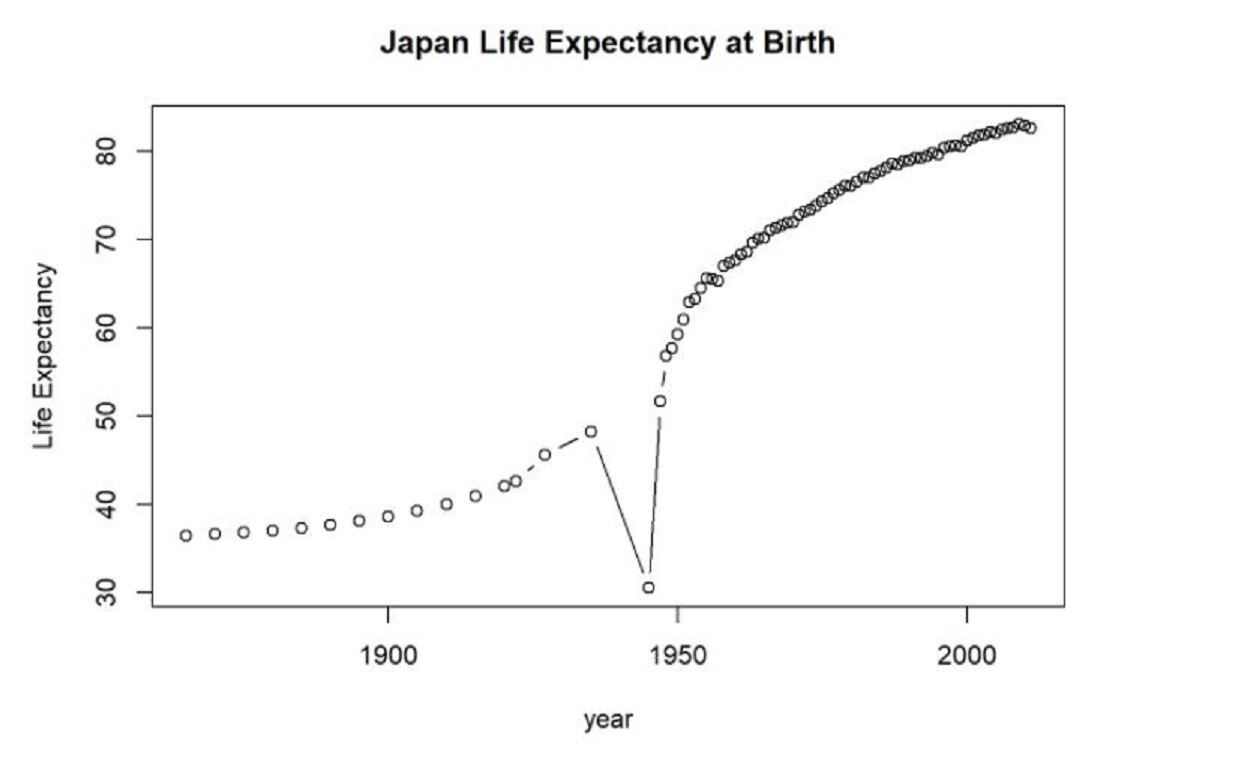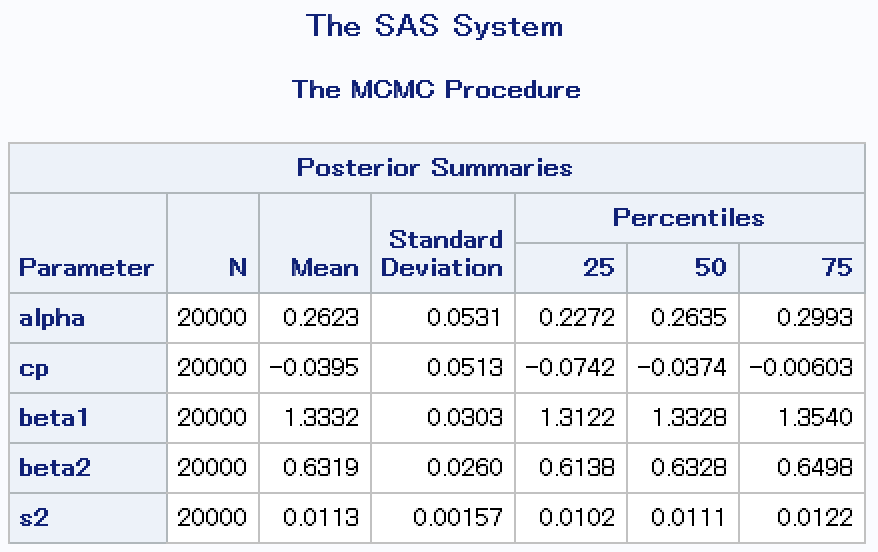## Introduction

Change-point analysis seeks to identify the point or points in a time series at which a change, or break in the trend, takes place. This technique is applicable to a wide variety of social science questions where the researcher is interested in the point of time at which a statistically significant change in the quantity being studied occurs.

Our tutorial demonstrates the application of change-point analysis to life expectancy data. Our World in Data provides time series of life expectancy at birth for many countries in the world over a long period of time. We selected two countries - the United States and Japan - on which to perform our change-point analysis. Life expectancy data for both these countries was available going back over a century. In the case of the United States, data was available for 1880, 1890, and every year from 1901 to 2011. For Japan, data was available approximately every five years from 1865 to 1945 and every year from 1947 to 2011.

Plotting the time series for each country, we can see that life expectancy has changed dramatically in both the United States and Japan over the domain of the data:While both time series clearly indicate an overall upward trend in life expectancy, less obvious is the specific year or years at which a change can be considered to have occurred. (Note that outliers are not necessarily change points, as change-point analysis is concerned with changes in the overall trend rather than individual point-to-point variations. However, certain change-point models may be more sensitive to outliers than others, as we’ll see below.)

Identifying these points of change in the trend is precisely what our analysis seeks to do. In total, we built three different change-point models: one in SAS using PROC MCMC (Markov Chain Monte Carlo), a second in R using the ‘bcp’ (Bayesian Change Point) package, and a third also in R using the ‘changepoint’ package. In the first SAS analysis, we assumed that there was only one underlying change point. The R packages allowed for multiple change points. Analysis and results are given below.

## Analysis and Results

### SAS: PROC MCMC

This SAS procedure performs Markov Chain Monte Carlo (MCMC) simulation to fit a wide range of Bayesian statistical models. Here we used it to create Bayesian change-point models for the life expectancy data.

The first stage involved preparing the data. We standardized the year and life expectancy variables prior to running the model.

``````/* read in the data */
proc import datafile="M:\Private\Stats506\project\life-expectancy.csv" dbms=CSV out=le_all;
run;

/* filter to just include selected country */
data le_usa;
set le_all;
where code = "USA";
*year = _N_;
run;

/* calculate summary statistics */
proc summary data=le_usa missing nway;
var year life_expectancy;
class code;
output out=le_usa_stats(drop=_TYPE_ _FREQ_)
mean(year) = meanyr std(year) = stdyr
mean(life_expectancy) = meanle std(life_expectancy) = stdle;
run;

/* standardize year and life_expectancy using summary statistics */
data le_usa_z;
merge le_usa(in=a) le_usa_stats(in=b);
by code;
if a;
x = (year-meanyr)/stdyr;
y = (life_expectancy - meanle)/stdle;

/* remove first two years when measurements were at 10-yr intervals */
if year in (1880,1890) then delete;
run;``````

We then used Markov Chain Monte Carlo simulation to fit a Bayesian change-point model, following closely the example given in the PROC MCMC documentation on the SAS website.

``````/* changepoint model using Markov Chain Monte Carlo */
proc mcmc data=le_usa_z outpost=postout seed=24860
ntu=1000 nmc=20000 /* use 20000 iterations after burn-in of 1000 */
statistics=summary;
ods select PostSummaries;
ods output PostSummaries=test1;

array beta;
parms alpha cp beta1 beta2;
parms s2;

prior cp ~ unif(-1.3, 1.1);
prior s2  ~ uniform(0, 5);
prior alpha beta:  ~ normal(0, v = 1e3);

j = 1 + (x >= cp);
mu = alpha + beta[j] * (x - cp);
model y ~ normal(mu, var=s2);
run;``````

Here are the resulting posterior summary statistics for the model parameters. Note that the standardized mean of the change point (cp) is \(-0.0395\). This corresponds to the gap in between the years 1953 and 1954.We can visualize the posterior distribution of the changepoint as follows. Once again, we rely heavily on the example from the SAS documentation.

``````data _null_;
set test1;
call symputx(parameter, mean);
run;

data b;
missing A;
input x1 @@;
if x1 eq .A then x1 = &cp;
if _n_ <= 2 then y1 = &alpha + &beta1 * (x1 - &cp);
else y1 = &alpha + &beta2 * (x1 - &cp);
datalines;
-3 A 1.5;
run;

proc kde data=postout;
univar cp / out=m1 (drop=count);
run;

data m1;
set m1;
density = (density / 3) - 4;
run;

data all;
set le_usa_z b m1;
rename x=Year_Standardized y=Life_Expectancy_Standardized;
run;``````

The posterior distribution of the change point appears roughly normal.